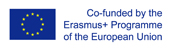This project has been funded with support from the European Commission.
This web site reflects the views only of the author, and the Commission cannot be held responsible for any use which may be made of the information contained therein.

Select language   >   IT EN RO PL LT

### Exponential growth

Subject taught
- Biology
- Math

Type of Product
- Videos

Language Skills Developed
- Interaction
- Listening
- Speaking
- Writing

Transferable/Scientific Skills Developed
Develop teamwork skills Develop listening, writing and speaking skills Know that exponential growth is unrealistic Know which are the environmental limits to exponential growth Know the meaning of logistic growth compare the shape of an exponential and a logistic growth

Description
This teaching resource takes into consideration general, linguistic and subject specific aims.
The general aims are the following:
- Make cross - curricular links combining maths with science
- Increase students’ motivation and interest in study by using clil methodology
- encourage students to develop the ability to think critically
- prepare students for life in a more internationalised society
- Develop the ability to think in English
- Let students work together to accomplish shared goals

The linguistic aims are the following:
- enable students to develop language skills which emphasize effective communication
- motivate students to learn English by using it for real practical purposes
- Promote the learning of a more extensive and varied vocabulary
- Allow students to practice understanding of a scientific talk
- Allow students to use the impersonal language to speculate about scientific topics

The subject specific aims are the following:
- understand the meaning of exponential growth
- understand the exponential rate of growth
- understand how maths can describe a natural process
- Understand that exponential growth is unrealistic because it assumes that a population can increase in number without any limitation
- understand which are the environmental limits to exponential growth
- understand the meaning of logistic growth
- Understand the shape of logistic function
- Understand the meaning of carrying capacity
- Know the concept of asymptote

Target group age: this activity is for students of the fourth year of high school from 17 to 18
Level of competence in English (CEFR) should be B1 at least
Time required to use the resource with the students: 30 minutes / 45 minutes
How to use it

The teacher could use a flipped classroom approach. The teacher could let the students watch the video as homework, then at school the teacher could divide the class into small group and ask them to answer some questions about exponential growth limitations.
The questions may be:
Can a population keep growing indefinitely?
Which factors can limit the population's growth?
What is carrying capacity?
Which are the differences between exponential and logistic growth?
Can you describe the shape of the logistic curve?
What mathematical concept lies behind the carrying capacity?
Possible difficulties for the students

- understand the difference between exponential and logistic growth
- Understand the meaning of carrying capacity
- talk in English about scientific topics
- Write answers to the questions in English